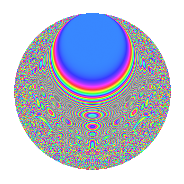# Properties

 Label 525.4.qLevel $525$ Weight $4$ Character orbit 525.q Rep. character $\chi_{525}(299,\cdot)$ Character field $\Q(\zeta_{6})$ Dimension $280$ Sturm bound $320$

# Related objects

## Defining parameters

 Level: $$N$$ $$=$$ $$525 = 3 \cdot 5^{2} \cdot 7$$ Weight: $$k$$ $$=$$ $$4$$ Character orbit: $$[\chi]$$ $$=$$ 525.q (of order $$6$$ and degree $$2$$) Character conductor: $$\operatorname{cond}(\chi)$$ $$=$$ $$105$$ Character field: $$\Q(\zeta_{6})$$ Sturm bound: $$320$$

## Dimensions

The following table gives the dimensions of various subspaces of $$M_{4}(525, [\chi])$$.

Total New Old
Modular forms 504 296 208
Cusp forms 456 280 176
Eisenstein series 48 16 32

## Trace form

 $$280q - 540q^{4} - 2q^{9} + O(q^{10})$$ $$280q - 540q^{4} - 2q^{9} - 2020q^{16} - 96q^{19} - 582q^{21} - 252q^{24} - 840q^{31} + 1008q^{36} - 120q^{39} + 1792q^{46} - 1108q^{49} + 198q^{51} - 4398q^{54} + 420q^{61} + 12752q^{64} + 168q^{66} + 1120q^{79} - 4342q^{81} + 9222q^{84} + 7740q^{91} - 9132q^{94} + 7758q^{96} + 764q^{99} + O(q^{100})$$

## Decomposition of $$S_{4}^{\mathrm{new}}(525, [\chi])$$ into newform subspaces

The newforms in this space have not yet been added to the LMFDB.

## Decomposition of $$S_{4}^{\mathrm{old}}(525, [\chi])$$ into lower level spaces

$$S_{4}^{\mathrm{old}}(525, [\chi]) \cong$$ $$S_{4}^{\mathrm{new}}(105, [\chi])$$$$^{\oplus 2}$$

## Hecke characteristic polynomials

There are no characteristic polynomials of Hecke operators in the database